Next: Image Formation by Plane Up: Paraxial Optics Previous: Image Formation by Concave

## Image Formation by Convex Mirrors

The definitions of the principal axis, centre of curvature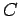, radius of curvature, and the vertex, of a convex mirror are analogous to the corresponding definitions for a concave mirror. When parallel light-rays strike a convex mirror they are reflected such that they appear to emanate from a single point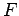located behind the mirror, as shown in Fig. 74. This point is called the virtual focus of the mirror. The focal length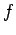of the mirror is simply the distance betweenand. As is easily demonstrated, in the paraxial approximation, the focal length of a convex mirror is half of its radius of curvature.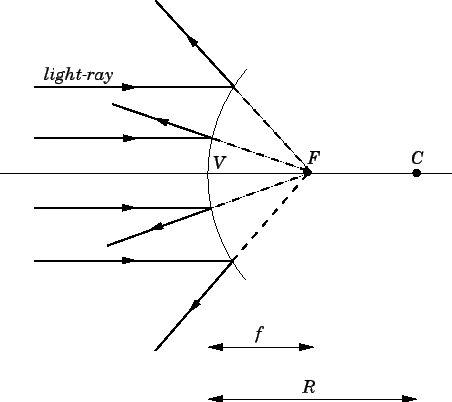There are, again, two alternative methods of locating the image formed by a convex mirror. The first is graphical, and the second analytical.

According to the graphical method, the image produced by a convex mirror can always be located by drawing a ray diagram according to four simple rules:

1. An incident ray which is parallel to the principal axis is reflected as if it came from the virtual focusof the mirror.
2. An incident ray which is directed towards the virtual focusof the mirror is reflected parallel to the principal axis.
3. An incident ray which is directed towards the centre of curvatureof the mirror is reflected back along its own path (since it is normally incident on the mirror).
4. An incident ray which strikes the mirror at its vertexis reflected such that its angle of incidence with respect to the principal axis is equal to its angle of reflection.
The validity of these rules in the paraxial approximation is, again, fairly self-evident.

In the example shown in Fig. 75, two rays are used to locate the image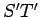of an object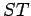placed in front of the mirror. It can be seen that the image is virtual, upright, and diminished.As is easily demonstrated, application of the analytical method to image formation by convex mirrors again yields Eq. (352) for the magnification of the image, and Eq. (358) for the location of the image, provided that we adopt the following sign convention. The focal lengthof a convex mirror is redefined to be minus the distance between pointsand. In other words, the focal length of a concave mirror, with a real focus, is always positive, and the focal length of a convex mirror, with a virtual focus, is always negative. Table 6 shows how the location and character of the image formed in a convex spherical mirror depend on the location of the object, according to Eqs. (352) and (358) (with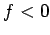).

Table 6: Rules for image formation by convex mirrors.
 Position of object Position of image Character of image At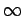AtVirtual, zero size BetweenandBetweenandVirtual, upright, diminished AtAtVirtual, upright, same size

In summary, the formation of an image by a spherical mirror involves the crossing of light-rays emitted by the object and reflected off the mirror. If the light-rays actually cross in front of the mirror then the image is real. If the light-rays do not actually cross, but appear to cross when projected backwards behind the mirror, then the image is virtual. A real image can be projected onto a screen, a virtual image cannot. However, both types of images can be seen by an observer, and photographed by a camera. The magnification of the image is specified by Eq. (352), and the location of the image is determined by Eq. (358). These two formulae can be used to characterize both real and virtual images formed by either concave or convex mirrors, provided that the following sign conventions are observed:

1. The height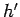of the image is positive if the image is upright, with respect to the object, and negative if the image is inverted.
2. The magnification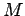of the image is positive if the image is upright, with respect to the object, and negative if the image is inverted.
3. The image distanceis positive if the image is real, and, therefore, located in front of the mirror, and negative if the image is virtual, and, therefore, located behind the mirror.
4. The focal lengthof the mirror is positive if the mirror is concave, so that the focal pointis located in front of the mirror, and negative if the mirror is convex, so that the focal pointis located behind the mirror.
Note that the front side of the mirror is defined to be the side from which the light is incident.Next: Image Formation by Plane Up: Paraxial Optics Previous: Image Formation by Concave
Richard Fitzpatrick 2007-07-14